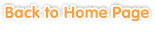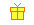Sample questions and games are shown below. Just click to start!• Chapter 1
From 0 to 10
••
Comparing Numbers and Sequencing••
Subtraction•• Chapter 2
Numbers 11 to 20
•
Counting•
Comparing Numbers and Sequencing•
Writing Numbers•
Addition Up to 20 without Regrouping••
Subtraction Up to 20 without Regrouping•
Subtraction Up to 20 with Regrouping•• Chapter 3
Problem Solving: Numbers up to 20• Chapter 4
Numbers 20 to 100
•
Counting by Groups•
Compare Numbers and Sequencing••
Ordinal Numbers•••
Adding 2-Digit Numbers with 1-Digit Numbers•
Subtracting 2-Digit Numbers with 10s•
Subtracting 2-Digit Numbers with 1-Digit Numbers•• Chapter 5
Problem Solving: Numbers up to 100• Chapter 6• Chapter 7
Patterns
•
Numbers•• Chapter 8
Classifying• Chapter 9
Plane and Solid Shapes• Chapter 10
Telling Time to Hour, Half Hour, and Quarter Hour• Chapter 11
Money
•
Names and Values of Coins and Bills Up to \$100•
Problem Solving• Chapter 12